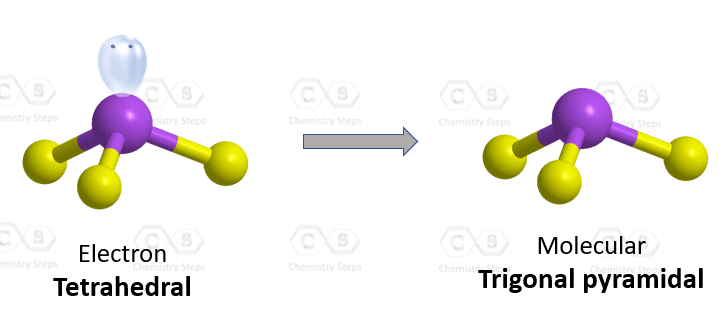## Examples

N is the central atom and there are 5 + 3×7 = 26 electrons. Each chlorine taking three lone pairs leaves the nitrogen with one lone pair:The central atom has 3 atoms and a lone pair (SN = 4). Therefore, the electron geometry is tetrahedral while the molecular geometry is trigonal pyramidal:Steric number 4 corresponds to sp3-hybridization where the idealized bond angles are 109.5o.

Check Also